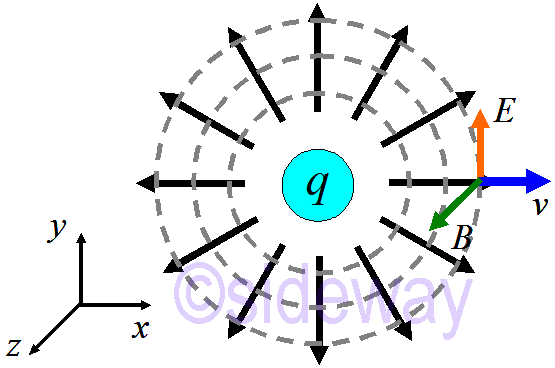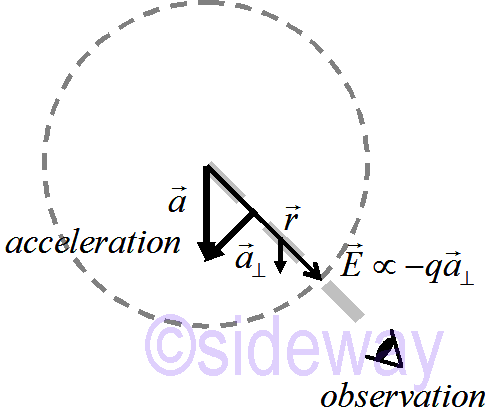output.to from Sideway
Draft for Information Only

# Content

` Maxwell's Equations with No Charges Wave Equations Solution of Wave Equation Electromagnetic Spectrum Accelerated Charges Why is the Sky Blue Source and Reference`

## Maxwell's Equations with No Charges

In the absence of sources, charges, current ```Gauss's Law: ∇⋅𝐸=1𝜀0𝜌⇒∇⋅𝐸=0 Gauss's Law (Magnetism): ∇⋅𝐵=0 ⇒∇⋅𝐵=0 Faraday's Law: ∇×𝐸=−∂𝐵∂𝑡 ⇒∇×𝐸=−∂𝐵∂𝑡 Ampere-Maxwell Law: ∇×𝐵=𝜇0𝐽+𝜀0∂𝐸∂𝑡 ⇒∇×𝐵=𝜇0𝜀0∂𝐸∂𝑡. ```

## Wave Equations

Once a wave starts, Both Faraday and Ampere equations keep going. Feed one equation into the other: ```∇×∇×𝐵=𝜇0𝜀0∂𝐸∂𝑡 ⇒∇×∇×𝐵=𝜇0𝜀0∂∂𝑡∇×𝐸=𝜇0𝜀0∂∂𝑡−∂𝐵∂𝑡=−1𝑐2∂2∂𝑡2𝐵 ⇒−∂2∂𝑥2+∂2∂𝑦2+∂2∂𝑧2𝐵=−1𝑐2∂2∂𝑡2𝐵 ⇒∂2∂𝑥2+∂2∂𝑦2+∂2∂𝑧2𝐵=1𝑐2∂2∂𝑡2𝐵 and similarly ⇒∂2∂𝑥2+∂2∂𝑦2+∂2∂𝑧2𝐸=1𝑐2∂2∂𝑡2𝐸 ``` In other words, both 𝐸 and 𝐵 are waving.

## Solution of Wave Equation

Let 𝐸 be wave of the form: 𝐸=𝐸0sin (𝑘𝑥−𝜔𝑡)𝑧
2∂𝑥2+2∂𝑦2+2∂𝑧2𝐸=2∂𝑥2𝐸=−𝑘2𝐸0sin (𝑘𝑥−𝜔𝑡)𝑧
1𝑐22∂𝑡2𝐸=−𝜔2𝑐2𝐸0sin (𝑘𝑥−𝜔𝑡)𝑧
If 𝜔=𝑐𝑘 then left and right terms are the same. And 𝐸=𝐸0sin (𝑘𝑥−𝜔𝑡)𝑧 is the wave solution of 𝐸.
For the differential form of Faraday's law, ×𝐸=−𝐵∂𝑡, let 𝐸 be wave of the form: 𝐸=𝐸0sin (𝑘𝑥−𝜔𝑡)𝑧, then
×𝐸=𝑥𝑦𝑧∂𝑥∂𝑦∂𝑧00𝐸𝑧=∂𝑦𝐸𝑧𝑥∂𝑥𝐸𝑧𝑦=−𝑘𝐸0cos (𝑘𝑥−𝜔𝑡)𝑦≡−𝐵∂𝑡
𝐵=𝐸0𝑘𝜔sin (𝑘𝑥−𝜔𝑡)𝑦=𝐸0𝑐sin (𝑘𝑥−𝜔𝑡)𝑦
Self-sustaining wave that can go on forever. 𝐸 and 𝐵 are perpendicular to each other, and to the direction of propagation: 𝑣𝐸×𝐵 where the magnitude of 𝑣 is 𝑐.

## Electromagnetic Spectrum

Gamma rays--X rays--Light(Ultra-Violet--Visible light--Infra-Red)--MicroWave--Radio(TV--FM--Amateur band--AM--Long Wave)

## Accelerated ChargesElectromagnetic pulse can propagate in space. Information about the charge's wiggle travels outward at the speed of light. The disturbance takes the shape of a sphere. 𝐸radial∼(1/𝑟^3);𝐸transverse∼(1/𝑟), therefore 𝐸transverse≫𝐸radial at large distance.
Transverse pulse propagates at speed of light. Since 𝐸(t), there must be 𝐵. Direction of 𝑣 is therefore given by: 𝐸×𝐵Magnitude can be derived from Gauss's law, first shown by Edward Purcell.
Field∼−𝑞𝑎
The direction of the transverse field is opposite to 𝑞𝑎. The electric field falls off at a rate 1/𝑟.

## Why is the Sky Blue

On the Moon, the sky looks black. Gas molecules scatter blue/violet light more than red light. Sky is blue for the same reason, the sunset is red.

## Source and Reference

ID: 200200802 Last Updated: 2/8/2020 Revision: 0Home 5

Management

HBR 3

Information

Recreation

Culture

Chinese 1097

English 337

Computer

Hardware 154

Software

Application 207

Latex 35

Manim 203

Numeric 19

Programming

Web 285

Unicode 504

HTML 65

CSS 63

SVG 9

ASP.NET 240

OS 422

Python 64

Knowledge

Mathematics

Algebra 84

Geometry 32

Calculus 67

Engineering

Mechanical

Rigid Bodies

Statics 92

Dynamics 37

Control

Natural Sciences

Electric 27